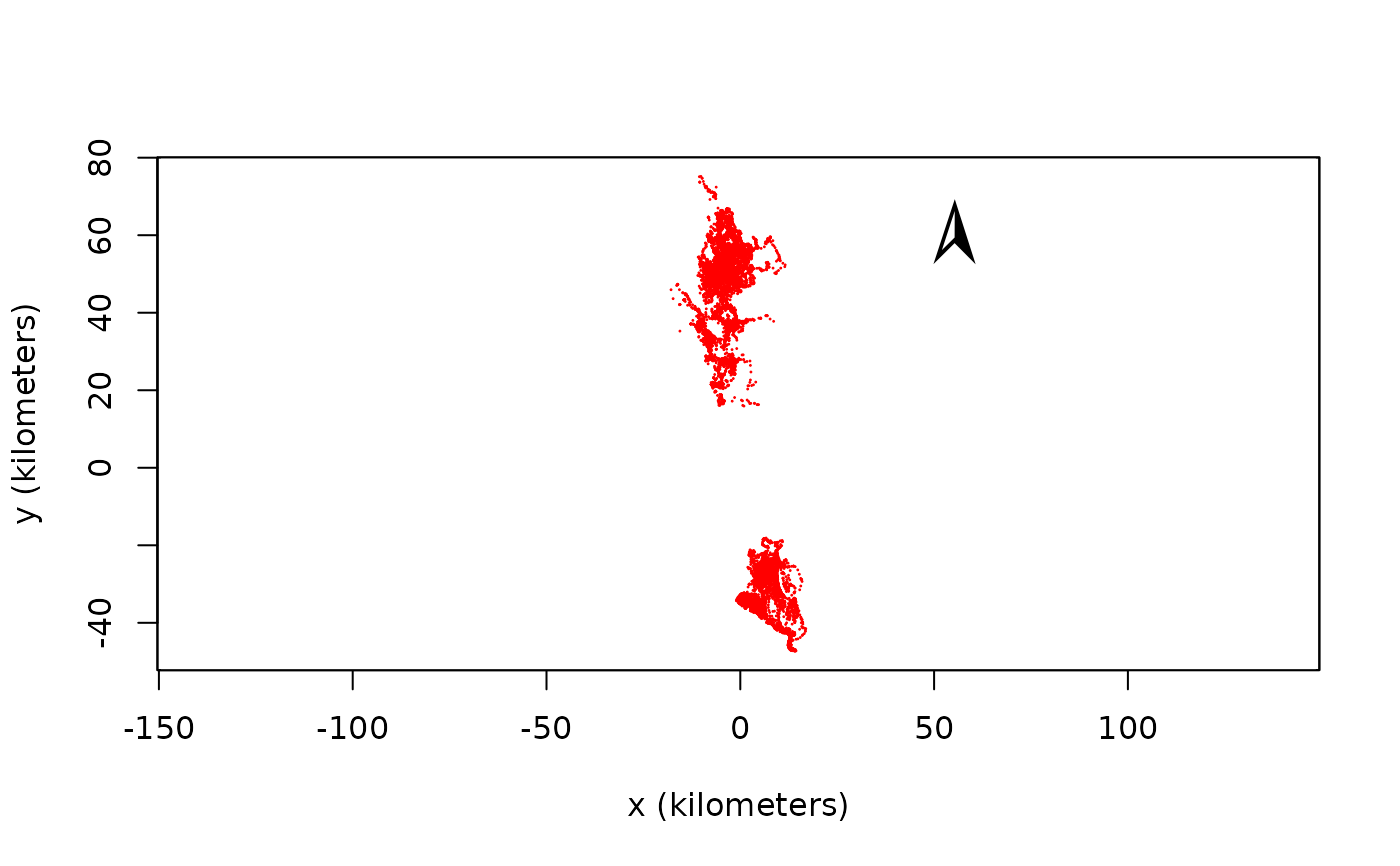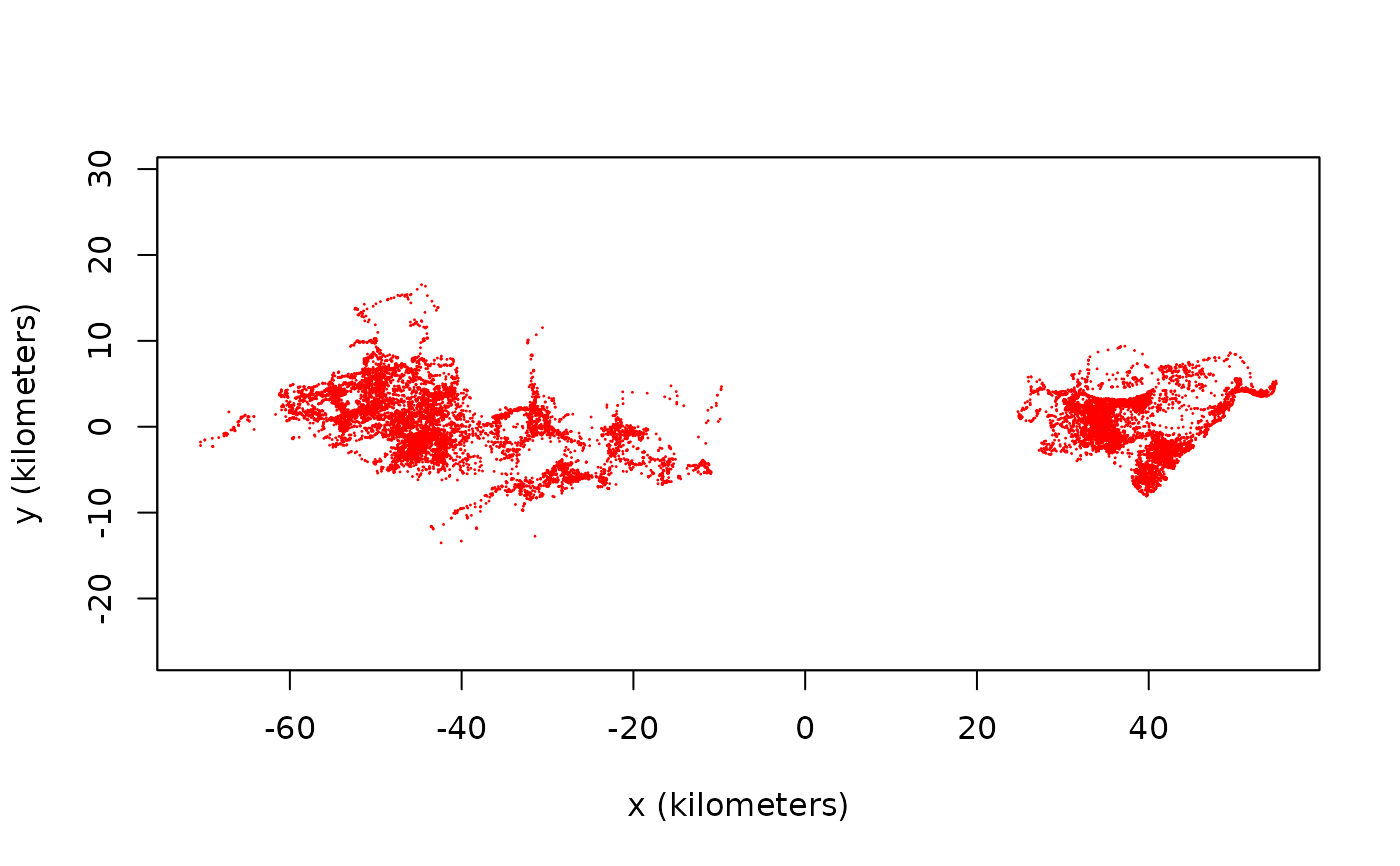Functions to manipulate the coordinate reference system (CRS) of ctmm objects

<!-- %projection(x,...) -->
# S4 method for telemetry
projection(x,asText=TRUE)

# S4 method for ctmm
projection(x,asText=TRUE)

# S4 method for UD
projection(x,asText=TRUE)

# S4 method for list
projection(x,asText=TRUE)

# S4 method for NULL
projection(x,asText=TRUE)

# S4 method for telemetry
projection(x) <- value

# S4 method for list
projection(x) <- value

# S3 method for telemetry
median(x,na.rm=FALSE,...)

compass(loc=NULL,cex=3,...) <!-- % fix this -->

## Arguments

x A telemetry, ctmm, or UD object. If TRUE, the projection is returned as text. Otherwise a CRS object is returned. Projection to apply. Can also be a data.frame of longitude-latitude foci. Not used. Arguments passed to Gmedian or text. Optional two-dimensional coordinates (in meters) at which to draw a north-facing compass needle. Relative size of compass.

## Details

projection(x) returns the projection information from ctmm object x, while projection(x) <- value applies the projection value to object x. median(x) returns the ellipsoidal geometric median of a telemetry object. compass(x,y) plots a north-pointing compass needle at the coordinates $$(x,y)$$.

## Author

C. H. Fleming

as.telemetry.

## Examples

# Load package and data
library(ctmm)
data(buffalo)

# Apply a 1-point projection that preserves North==up
projection(buffalo) <- median(buffalo)
plot(buffalo)
#> DOP values missing. Assuming DOP=1.#> DOP values missing. Assuming DOP=1.#> DOP values missing. Assuming DOP=1.#> DOP values missing. Assuming DOP=1.#> DOP values missing. Assuming DOP=1.#> DOP values missing. Assuming DOP=1.compass()# Apply a 2-point projection safer for elongated disributions
projection(buffalo) <- median(buffalo,k=2)
# This is the default projection for ctmm
plot(buffalo)
#> DOP values missing. Assuming DOP=1.#> DOP values missing. Assuming DOP=1.#> DOP values missing. Assuming DOP=1.#> DOP values missing. Assuming DOP=1.#> DOP values missing. Assuming DOP=1.#> DOP values missing. Assuming DOP=1.compass()